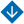State Estimation Methods for fuzzy integral models. Part II: Least Squares Method and Direct Variational Calculus Methods
•About Advisory & Editorial Boards Aims & Scope Publication Ethics Review Process Archive Contact Information

#State Estimation Methods for fuzzy integral models. Part II: Least Squares Method and Direct Variational Calculus Methods

Demenkov, N.P., Mikrin, E.A. , and Mochalov, I.A. State Estimation Methods for fuzzy integral models. Part II: Least Squares Method and Direct Variational Calculus Methods
Abstract. This paper considers the least squares method (LSM) and its modifications for estimating the states of fuzzy integral models, namely, LSM with numerical integration, recurrent and nonlinear LSM, and fuzzy LSM, which is based on fuzzy rules for finding diagonal elements of the weight matrix in generalized LSM. Some examples of fuzzy systems of linear equations (FSLEs) arising in state estimation problems for fuzzy integral models are given and solved. The fuzzy Galerkin method is implemented for the approximate state estimation of a fuzzy integral model. This method leads to a fully FSLE. The emergence of “strong” and “weak” systems is explained using an illustrative example. Chebyshev quadrature methods and sinc functions for the approximate structural estimation of fuzzy integral models are considered. As noted in the paper, the same methodology can be applied to develop other algorithms for estimating fuzzy integral models based on the following methods: residuals, collocation, energy, Ritz, Courant, etc.

Keywords: fuzzy least squares method, fuzzy Galerkin method, fuzzy Chebyshev method, fuzzy sinc method.

PDF (English)